# x86

Jul. 5, 2015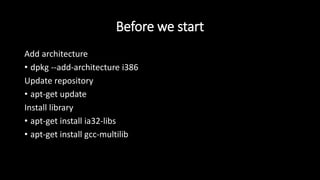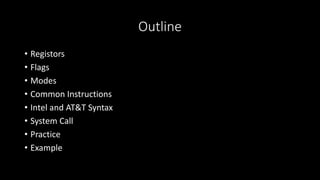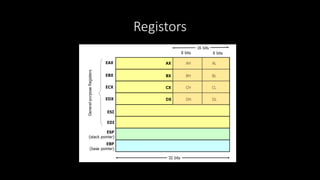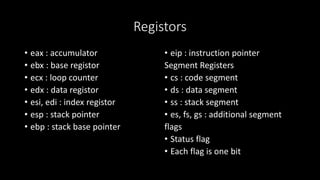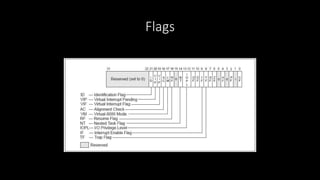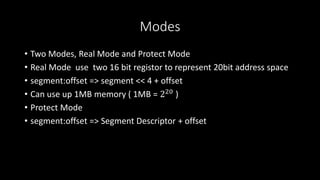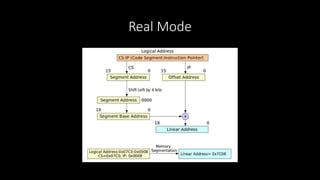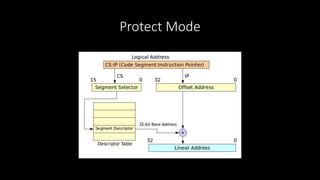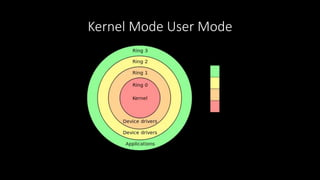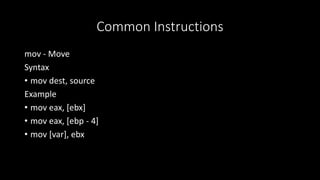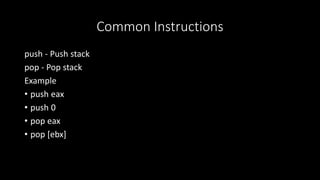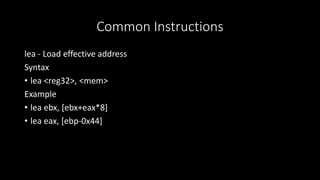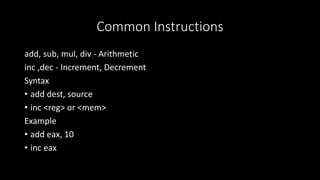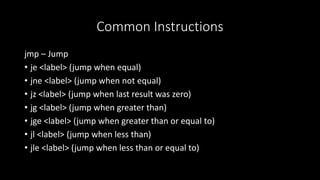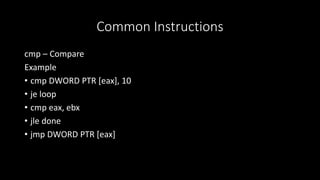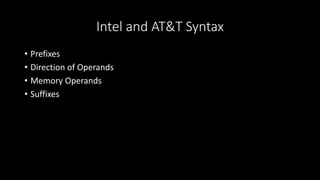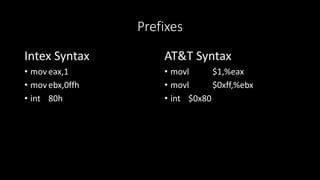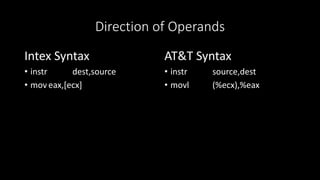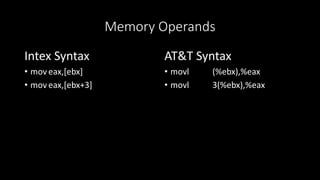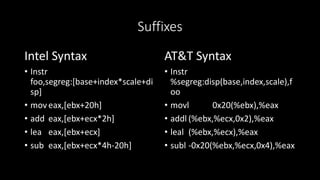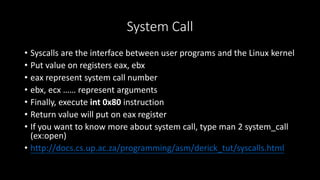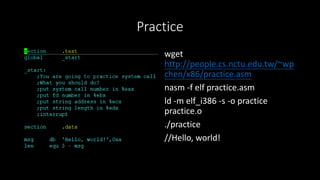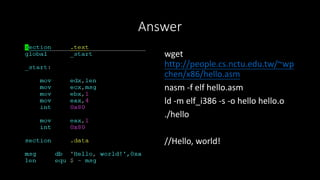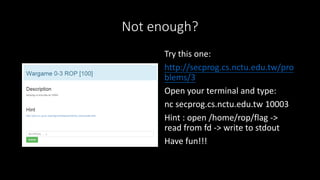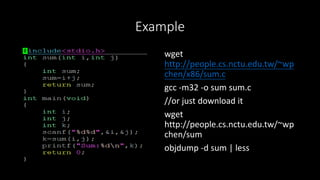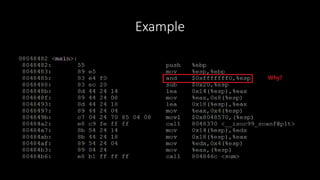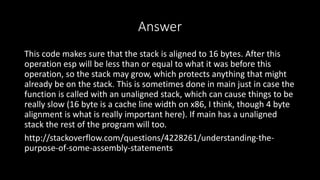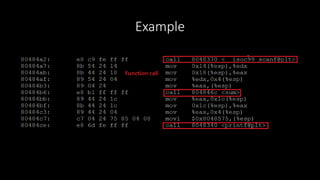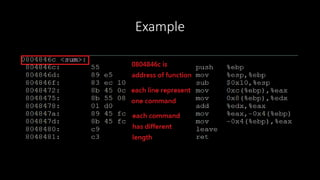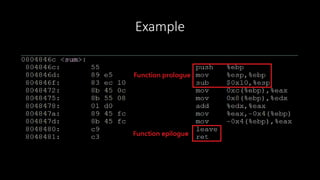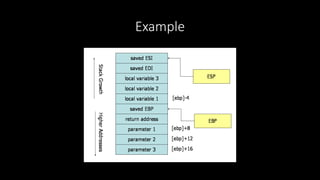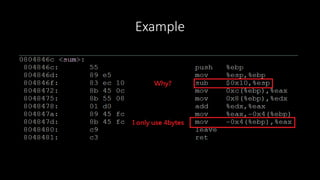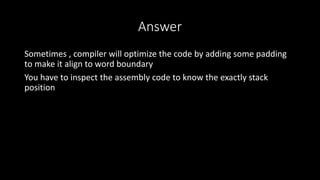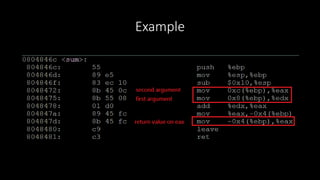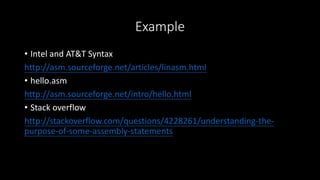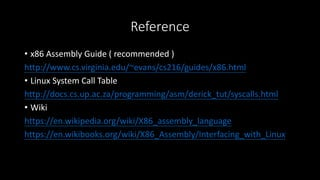1 of 38

### x86

• 1. x86 bananaapple
• 2. Before we start Add architecture • dpkg --add-architecture i386 Update repository • apt-get update Install library • apt-get install ia32-libs • apt-get install gcc-multilib
• 3. Who am I? • ID : bananaapple • 學校科系 : 交通大學資工系 • 年級 : 大三升大四 • 目前為 Bamboofox 中的一員
• 4. Outline • Registors • Flags • Modes • Common Instructions • Intel and AT&T Syntax • System Call • Practice • Example
• 5. Registors
• 6. Registors • eax : accumulator • ebx : base registor • ecx : loop counter • edx : data registor • esi, edi : index registor • esp : stack pointer • ebp : stack base pointer • eip : instruction pointer Segment Registers • cs : code segment • ds : data segment • ss : stack segment • es, fs, gs : additional segment flags • Status flag • Each flag is one bit
• 7. Flags
• 8. Modes • Two Modes, Real Mode and Protect Mode • Real Mode use two 16 bit registor to represent 20bit address space • segment:offset => segment << 4 + offset • Can use up 1MB memory ( 1MB = 220 ) • Protect Mode • segment:offset => Segment Descriptor + offset
• 9. Real Mode
• 10. Protect Mode
• 11. Kernel Mode User Mode
• 12. Common Instructions mov - Move Syntax • mov dest, source Example • mov eax, [ebx] • mov eax, [ebp - 4] • mov [var], ebx
• 13. Common Instructions push - Push stack pop - Pop stack Example • push eax • push 0 • pop eax • pop [ebx]
• 14. Common Instructions lea - Load effective address Syntax • lea <reg32>, <mem> Example • lea ebx, [ebx+eax*8] • lea eax, [ebp-0x44]
• 15. Common Instructions add, sub, mul, div - Arithmetic inc ,dec - Increment, Decrement Syntax • add dest, source • inc <reg> or <mem> Example • add eax, 10 • inc eax
• 16. Common Instructions jmp – Jump • je <label> (jump when equal) • jne <label> (jump when not equal) • jz <label> (jump when last result was zero) • jg <label> (jump when greater than) • jge <label> (jump when greater than or equal to) • jl <label> (jump when less than) • jle <label> (jump when less than or equal to)
• 17. Common Instructions cmp – Compare Example • cmp DWORD PTR [eax], 10 • je loop • cmp eax, ebx • jle done • jmp DWORD PTR [eax]
• 18. Intel and AT&T Syntax • Prefixes • Direction of Operands • Memory Operands • Suffixes
• 19. Prefixes Intex Syntax • mov eax,1 • mov ebx,0ffh • int 80h AT&T Syntax • movl \$1,%eax • movl \$0xff,%ebx • int \$0x80
• 20. Direction of Operands Intex Syntax • instr dest,source • mov eax,[ecx] AT&T Syntax • instr source,dest • movl (%ecx),%eax
• 21. Memory Operands Intex Syntax • mov eax,[ebx] • mov eax,[ebx+3] AT&T Syntax • movl (%ebx),%eax • movl 3(%ebx),%eax
• 22. Suffixes Intel Syntax • Instr foo,segreg:[base+index*scale+di sp] • mov eax,[ebx+20h] • add eax,[ebx+ecx*2h] • lea eax,[ebx+ecx] • sub eax,[ebx+ecx*4h-20h] AT&T Syntax • Instr %segreg:disp(base,index,scale),f oo • movl 0x20(%ebx),%eax • addl (%ebx,%ecx,0x2),%eax • leal (%ebx,%ecx),%eax • subl -0x20(%ebx,%ecx,0x4),%eax
• 23. System Call • Syscalls are the interface between user programs and the Linux kernel • Put value on registers eax, ebx • eax represent system call number • ebx, ecx …… represent arguments • Finally, execute int 0x80 instruction • Return value will put on eax register • If you want to know more about system call, type man 2 system_call (ex:open) • http://docs.cs.up.ac.za/programming/asm/derick_tut/syscalls.html
• 24. Practice wget http://people.cs.nctu.edu.tw/~wp chen/x86/practice.asm nasm -f elf practice.asm ld -m elf_i386 -s -o practice practice.o ./practice //Hello, world!
• 25. Answer wget http://people.cs.nctu.edu.tw/~wp chen/x86/hello.asm nasm -f elf hello.asm ld -m elf_i386 -s -o hello hello.o ./hello //Hello, world!
• 26. Not enough? Try this one: http://secprog.cs.nctu.edu.tw/pro blems/3 Open your terminal and type: nc secprog.cs.nctu.edu.tw 10003 Hint : open /home/rop/flag -> read from fd -> write to stdout Have fun!!!
• 27. Example wget http://people.cs.nctu.edu.tw/~wp chen/x86/sum.c gcc -m32 -o sum sum.c //or just download it wget http://people.cs.nctu.edu.tw/~wp chen/sum objdump -d sum | less
• 28. Example
• 29. Answer This code makes sure that the stack is aligned to 16 bytes. After this operation esp will be less than or equal to what it was before this operation, so the stack may grow, which protects anything that might already be on the stack. This is sometimes done in main just in case the function is called with an unaligned stack, which can cause things to be really slow (16 byte is a cache line width on x86, I think, though 4 byte alignment is what is really important here). If main has a unaligned stack the rest of the program will too. http://stackoverflow.com/questions/4228261/understanding-the- purpose-of-some-assembly-statements
• 30. Example
• 31. Example
• 32. Example
• 33. Example
• 34. Example
• 35. Answer Sometimes , compiler will optimize the code by adding some padding to make it align to word boundary You have to inspect the assembly code to know the exactly stack position
• 36. Example
• 37. Example • Intel and AT&T Syntax http://asm.sourceforge.net/articles/linasm.html • hello.asm http://asm.sourceforge.net/intro/hello.html • Stack overflow http://stackoverflow.com/questions/4228261/understanding-the- purpose-of-some-assembly-statements
• 38. Reference • x86 Assembly Guide ( recommended ) http://www.cs.virginia.edu/~evans/cs216/guides/x86.html • Linux System Call Table http://docs.cs.up.ac.za/programming/asm/derick_tut/syscalls.html • Wiki https://en.wikipedia.org/wiki/X86_assembly_language https://en.wikibooks.org/wiki/X86_Assembly/Interfacing_with_Linux